# NCERT Solution Exercise 7.1 class 10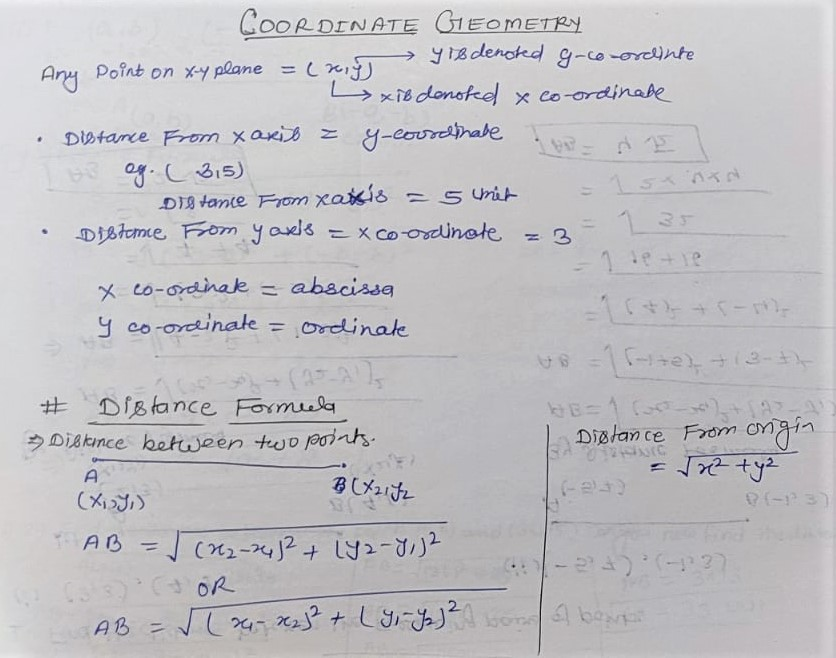Q1. Find the distance between the Following pairs of points:

(i) (2,3),(4,1)

Solution:

Let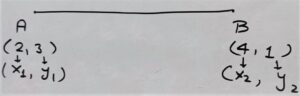By Distance Formula

A B = √(x2 – x1)² + (y2 – y1

⇒ A B = √(4 – 2)² + (1 – 3)²

⇒ A B = √(2)² + (-2)²

⇒ A B = √4 + 4

⇒ A B = √8

⇒ A B = 2√2

(ii) (-5,7),(-1,3)

Solution:

Let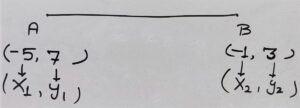A B = √(x2 – x1)² + (y2 – y1

⇒ A B = √(-1 + 5)² + (3 – 7)²

⇒ A B = √(4)² + (-4)²

⇒ A B = √16 + 16

⇒ A B = √32

⇒ A B = 4√2

(iii) (a, b),(-a, -b)Solution:

A B = √(x2 – x1)² + (y2 – y1

⇒ A B = √(-a – a)² + (-b – b)²

⇒ A B = √(-2a )² + (-2b)²

⇒ A B = √4a² + 4b²

⇒ A B = √4(a² + b²)

⇒ A B = 2√(a² + b²)

Q.2 Find the distance between the points (0,0) and (36,15).

A B = √(x2 – x1)² + (y2 – y1

⇒ A B = √(36 – 0)² + (15 – 0)²

⇒ A B = √(36)² + √(15)²

⇒ A B = √1296+225

⇒ A B = √1521

⇒ A B = √3×3×13×13

⇒ A B = 3×13

⇒ A B = 39 units

Q.3 Determine if the points (1, 5) (2, 3) and (-2, -11) are collinear.

Solution:

Let A(1, 5) B(2, 3) and C(-2, -11)

Distance A B = √(x2 – x1)² + (y2 – y1

⇒ A B = √(2-1)² + (3 – 5)²

⇒ A B = √ (1)² + (-2)²

⇒ A B = √1+4

⇒ A B = √5 units

Distance B C = √(x2 – x1)² + (y2 – y1

⇒ B C = √(-2 – 2)² + (-11 – 3)²

⇒ B C = √(-4)² + (-14)²

⇒ B C = √16 + 196

⇒ B C = √212 units

A C = √(x2 – x1)² + (y2 – y1

⇒ A C = √(-2 -1)² + (-11 -5)²

⇒ A C = √(-3)² + (-16)²

⇒ A C = √ 9 + 256

⇒ A C = √265

From above we can say that not sum of any two is equal to third therefore these points are not co-linear

Q.4 Check whether (5, -2), (6, 4) and (7, -2) are the vertices of an isosceles triangle.

Solution:

Let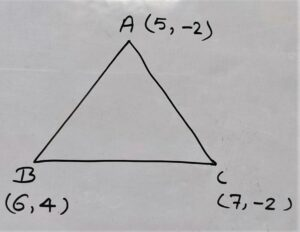Distance A B = √(x2 – x1)² + (y2 – y1

⇒ A B = √(6 – 5)² + (4 + 2)²

⇒ A B = √(1)² + (6)²

⇒ A B = √1+36

⇒ A B = √37

Distance B C = √(x2 – x1)² + (y2 – y1

⇒ B C = √(7 – 6)² + (-2 – 4)²

⇒ B C = √(1)² + (-6)²

⇒ B C = √1+36

⇒ B C = √37

Distance A C = √(x2 – x1)² + (y2 – y1

⇒ A C = √(7 – 5)² + (-2 + 2)²

⇒ A C = √(2)² + (0)²

⇒ A C = √4

⇒ A C = 2units

Hence

A B = B C ≠ A C

Now If two sides are equal then it is isosceles Triangle

∴ Δ ABC is Isosceles Triangle

Q.5 In a classroom, 4 friends are seated at the points A, B, C and D as shown in Fig. 7.8. Champa and Chameli walk into the class and after observing for a few minutes Champa asks Chameli, “Don’t you think ABCD is a square?” Chameli disagrees. Using distance formula, find which of them is correct.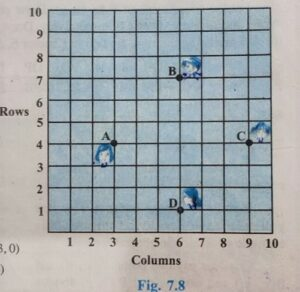Solution:

According to figure coordinates of following points are

A = (3,4),

B = (6,7)

C = (9,4)

D = (6,1)

Now we will check for square by distance formula

A (3,4)•——————–•B(6,7)

Distance A B = √(x2 – x1)² + (y2 – y1

⇒ Distance A B = √(6 – 3)² + (7 – 4)²

⇒ Distance A B = √(3)² + (3)²

⇒ Distance A B = √9+9

⇒ Distance A B = √18

B (6,7)•——————–•C(9,4)

Distance B C = √(x2 – x1)² + (y2 – y1

Distance B C = √(9 – 6)² + (4 -7)²

Distance B C = √(3)² + (-3)²

Distance B C = √(9+9)

Distance B C = √18

C (9,4)•——————–•D(6,1)

Distance C D = √(x2 – x1)² + (y2 – y1

⇒ Distance C D = √(6-9)² + (1-4)²

⇒ Distance C D = √(-3)² + (-3)²

⇒ Distance C D = √(9+9)

⇒ Distance C D = √18

A(3,4)•——————–•D(6,1)

Distance A D = √(x2 – x1)² + (y2 – y1

⇒ Distance A D = √(6 – 3)² + (1 – 4)²

⇒ Distance A D = √(3)² + (-3)²

⇒ Distance A D = √9+9

⇒ Distance A D = √18

A(3,4)•——————–•C(9,4)

Distance A C = √(x2 – x1)² + (y2 – y1

⇒ Distance A C = √(9 – 3)² + (4 – 4)²

⇒ Distance A C = √(6)² + (0)²

⇒ Distance A C = √36

⇒ Distance A C = 6

B(6,7)•——————–•D(6,1)

Distance B D = √(x2 – x1)² + (y2 – y1

⇒ Distance B D = √(6-6)² + (1-7)²

⇒ Distance B D = √(0)² + (-6)²

⇒ Distance B D = √36

⇒ Distance B D = 6

AB = BC = CD = AD = √18 and Diagonal A C = B D = 6

Hence all sides are equal and diagonals also equal

∴ Chameli was right, it is a square

Q.6 Name the type of quadrilateral formed , if only, by the following points, and give reasons for your answer:

(i) (-1, -2) (1, 0) (-1, 2) (-3, 0)

Let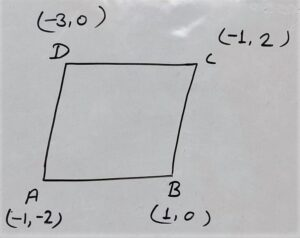Distance A B = √(x2 – x1)² + (y2 – y1

⇒ A B = √(1 + 1)² + (0 + 2)²

⇒ A B = √(2)² + (2)²

⇒ A B = √4 +4

⇒ A B = √8

⇒ A B = 2√2

Distance B C = √(-1 – 1)² + (2 – 0)²

⇒ B C = √(-2)²+(2)²

⇒ B C = √4 + 4

⇒ B C = √8

⇒ B C = 2√2

Distance C D = √(x2 – x1)² + (y2 – y1

⇒ C D = √(-3 + 1)² + (0 – 2)²

⇒ C D = √(-2)² + (-2)²

⇒ C D = √4 + 4

⇒ C D = √8

⇒ C D = 2√2

Distance A D = √(x2 – x1)² + (y2 – y1

⇒ A D = √(x2 – x1)² + (y2 – y1

⇒ A D = √(-3 + 1)² + (0 + 2)

⇒ A D = √(-2)² + (2)²

⇒ A D = √4+4

⇒ A D = √8

⇒ A D = 2√2

From Above

A B = B C = C D = A D = 2√2

Diagonal A(-1, -2) and C(-1, 2)

Diagonal A C =√(-1+1)² + (2+2)²

⇒ A C = √(0)² + (4)²

⇒ A C = √16

⇒ A C = 4 units

Diagonal B D = √(-3-1)²+(0-0)²

⇒  B D = √(-4)²

⇒ B D = √16

⇒ B D = 4

Diagonal BD = Diagonal AC

Hence All sides are equal and Diagonal Also equal therefore it is the coordinates of Square.

(ii) (-3, 5) (3, 1) (0, 3) (-1, -4)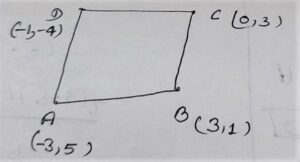According to figure

Distance A B = √(x2 – x1)² + (y2 – y1

⇒ A B = √(3 + 3)² + (1 – 5)²

⇒ A B = √(6)² + (- 4)²

⇒ A B = √(36 + 16)²

⇒ A B = √(52)²

⇒ A B = 2√13units

Distance B C = √(x2 – x1)² + (y2 – y1

⇒ Distance B C = √(0 – 3)² + (3 – 1)²

⇒ Distance B C = √(-3)² + (2)²

⇒ Distance B C = √(9+4)

⇒ Distance B C = √13units

Distance C D = √(x2 – x1)² + (y2 – y1

⇒ Distance C D = √(-1 – 0)² + (3+4)²

⇒ Distance CD = √(-1 )² + (7)²

⇒ Distance CD = √1 + 49

⇒ Distance CD = √50

⇒ Distance CD = 5√2units

Distance A D = √(x2 – x1)² + (y2 – y1

⇒ Distance A D = √(-1 + 3)² + (-4 – 5)²

⇒ Distance A D = √(2)² + (-9)²

⇒ Distance A D = √4 + 81

⇒ Distance A D = √85 units

Distance A C = √(x2 – x1)² + (y2 – y1

⇒ Distance A C = √(0+3)² + (3-5)²

⇒ Distance A C = √(3)² + (-2)²

⇒ Distance A C = √9 + 4

⇒ Distance A C = √13

Here

B C + A C = A B

√13 + √13 = 2√13

ABC are colinear

Hence Given Co-ordinates are not form a quadrilateral

(iii) (4, 5) (7, 6) (4, 3) (1, 2)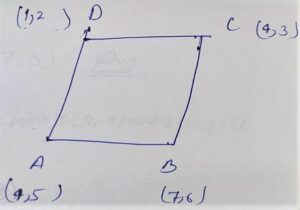Let A (4, 5) , B (7, 6) , C (4, 3) and D(1, 2)

Distance A B = √(x2 – x1)² + (y2 – y1

⇒ Distance A B = √(7 – 4)² + (6 – 5)²

⇒ Distance A B = √(3)² + (1)²

⇒ Distance A B = √9+1

⇒ Distance A B = √10 units

Distance B C = √(x2 – x1)² + (y2 – y1

⇒ Distance B C  = √(4 – 7)² + (3 – 6)²

⇒ Distance B C = √(-3)² + (-3)²

⇒ Distance B C = √(9 + 9)

⇒ Distance B C = √18

⇒ Distance B C = 3√2 units

Distance C D =√(x2 – x1)² + (y2 – y1

⇒ Distance C D = √(1 – 4)² + (2 – 3)²

⇒ Distance C D = √(-3)² + (-1)²

⇒ Distance C D = √(9+1)

⇒ Distance C D = √10

Distance A D = √(x2 – x1)² + (y2 – y1

⇒ Distance A D = √(1 – 4)² + (2 – 5)

⇒ Distance A D = √(-3)² + (-3)²

⇒ Distance A D = √(9+9)

⇒ Distance A D = √19

⇒ Distance A D = 3√2units

Diagonal A (4,5) and C(4,3)

Distance A C = √(x2 – x1)² + (y2 – y1

⇒ Distance A C = √(4 – 4)² + (3 – 5)²

⇒ Distance A C = √(0)² + (-2)²

⇒ Distance A C = √4

⇒ Distance A C = 2 units

B(7,6) •——————• D(1,2)

Distance B D = √(x2 – x1)² + (y2 – y1

⇒ Distance B D = √(1 – 7)² + (2 – 6)²

⇒ Distance B D = √(-6)² + (- 4)²

⇒ Distance B D = √(36+16)

⇒ Distance B D = √(52)

⇒ Distance B D = √2×2×13

⇒ Distance B D = 2√13units

Now

A B = C D = √10 and B C = A D = 3√2 but Diagonal A C ≠ B D

Hence ABCD is a parallelogram.

Q.7 Find the point in the x- axis which is equidistant from (2,-5) and (-2,9).

Solution:

Let the coordinate in X- axis be (x,0), and A (2,-5) and B(-2, 9)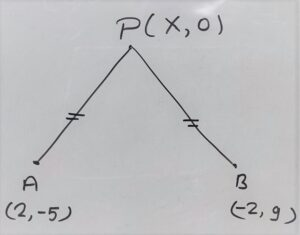Distance P A = Distance P B

√(x2 – x1)² + (y2 – y1)² = √(x2 – x1)² + (y2 – y1

⇒ √(2 – x)² + (-5 – 0)² = √(-2 – x)² + (9 – 0)²

⇒ (2 – x)² + (-5 – 0)² = (-2 – x)² + (9 – 0)²

⇒ (2)² – 2×2×x + x² + (-5)² = (-2)² -2×-2×x + x²

⇒ 4 – 4x + x² + 25 = 4 + 4x + x²

⇒  4 – 4x + x² + 25 – 4 – 4x – x² = 0

⇒ -8x + 25 = 0

⇒ 8x = – 25

⇒ x = -25/8

⇒ x = -7

Hence the coordinate on x-axis = (-7,0)

Q.8 Find the values of y for which the distance between the points P (2,-3) and Q (10,y) is 10 units.

Solution:

By Distance Formula

Distance P Q = 10

√(x2 – x1)² + (y2 – y1)² = Distance P Q

⇒ √(10 – 2)² + (y +3)² = 10

squaring both side

⇒ (8)² + (y +3)² = 10²

⇒ 64 + y² + 2×y×3  + 3² = 100

⇒ 64 + y² + 6y + 9 = 100

⇒ 73 + y² + 6y = 100

⇒ 73 + y² + 6y – 100 = 0

⇒ y² + 6y – 27 = 0

⇒ y² + (9-3)y – 27 = 0

⇒ y² + 9y – 3y – 27 = 0

⇒ y(y + 9) – 3(y – 9) = 0

⇒ (y + 9)(y – 3) = 0

y + 9 = 0 ; y – 3 = 0

y = -9 ; y = 3

y = -9,3

Q.9 If Q (0, 1) is equidistance from P(5, -3) and R(x, 6).Find the value of x also find the distances Q R and P R.

Solution:

Let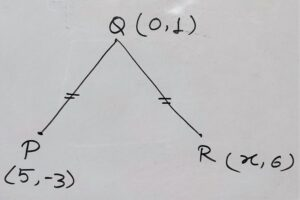According to Question

Q P = Q R

⇒ √(x2 – x1)² + (y2 – y1)² = √(x2 – x1)² + (y2 – y1

⇒ √(5 – 0)² + (-3 – 1)² = √(x – 0)² + (6 – 1)²

⇒ √(5) ² + (-4)² = √(x)² + (5)²

⇒ √(25+16) = √(x²+25)

squaring both side

⇒ 41 = x² + 25

⇒ 41 – 25 = x²

⇒ 16 = x²

⇒ ±4 = x

Hence x = 4,-4

Q (0, 1) •——————• R(5,-3)

Distance Q P = √(x2 – x1)² + (y2 – y1

⇒ Distance Q P = √(5 – 0)² + (-3 – 1)²

⇒ Distance Q P = √(5) ² + (-4)²

⇒ Distance Q P = √(25+16)

⇒ Distance Q P = √(41) units

If x = -4

Q (0,1)  ⋅———————–⋅ R (-4,6)

Q R = √(x2 – x1)² + (y2 – y1

⇒ Q R = √(-4 – 0)² + (6 – 1)²

⇒ Q R = √(-4 )² + (5)²

⇒ Q R = √(16+25)

⇒ Q R = √41

P(5, -3) ⋅———————–⋅R(-4, 6)

P R = √(x2 – x1)² + (y2 – y1

⇒ P R = √(-4 – 5)² + (6 + 3 )²

⇒ P R = √(-1)² + (9)²

⇒ P R = √1 + 81

⇒ P R = √82

If x = 4 then

P(5, -3)  ⋅———————–⋅ Q(4,6)

P Q = √(4-5)² + (6+3)

⇒ P Q = √(-1)² + (9)²

⇒ P Q = √(1+81)

⇒ P Q = √82

Q.10 Find a relationship between x and y such that the point (x, y) is equidistant from the point (3,6) and (-3,4).

Given:

Let P(x, y) Equidistance from Q(3,6) and R(-3,4)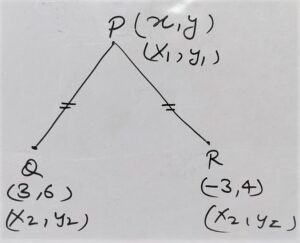Distance P Q = Distance P R

⇒ √(x2 – x1)² + (y2 – y1)² = √(x2 – x1)² + (y2 – y1

⇒ √(3 – x)² + (6 – y)² = √(-3 – x)² + (4 – y)²

Squaring both side

⇒ (3 – x)² + (6 – y)² = (-3 – x)² + (4 – y)²

⇒ 3² – 2×3×x + x² + 6² – 2×6×y + y² = (-3)² – 2×-3×x + x² + 4² – 2×4×y +y²

⇒ 9 – 6x + x² + 36 – 12y + y² = 9 + 6x + x² + 16 – 8y + y²

⇒ 9 – 6x + x² + 36 – 12y + y² -9 -6x – x² – 16 + 8y – y² = 0

⇒ -12x – 4y + 20 = 0

⇒ -4(3x + y + 5) = 0

⇒ 3x + y + 5 = 0

⇒ 3x + y = -5